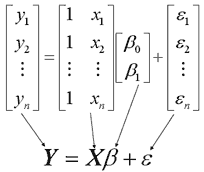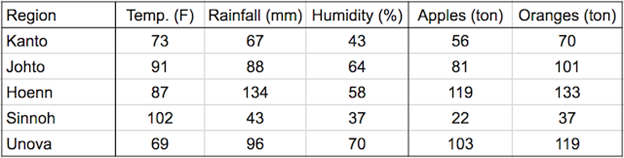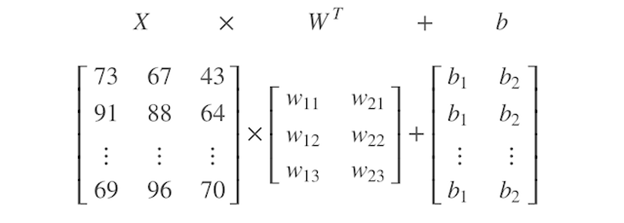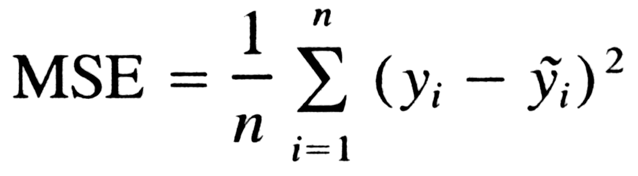1669771276

# How to Do Linear Regression in Pytorch

In this Pytorch article we will learn How to do Linear Regression in Pytorch. Linear regression is a statistical technique for estimating the relationship between two variables. A simple example of linear regression is to predict the height of someone based on the square root of the person’s weight (that’s what BMI is based on). To do this, we need to find the slope and intercept of the line. The slope is how much one variable changes with the change in other variable by one unit. The intercept is where our line crosses with the y-axis.

Let’s use the simple linear equation y=wx+b as an example. The output variable is y, while the input variable is x. The slope and y-intercept of the equation are represented by the letters w and b, hence referring them as the equation’s parameters. Knowing these parameters allows you to forecast the outcome y for any given value of x.

Now that you have learnt some basics of the simple linear regression, let’s try to implement this useful algorithm in the PyTorch framework. Here, we’ll focus on a few points described as follows:

• What is Linear Regression and how it can be implemented in PyTorch.
• How to import linear class in PyTorch and use it for making predictions.
• How we can build custom module for a linear regression problem, or for more complex models in the future.

So let’s get started.

## Overview

This tutorial is in three parts; they are

• Preparing Tensors
• Using Linear Class from PyTorch
• Building a Custom Linear Class

## Preparing Tensors

Note that in this tutorial we’ll be covering one-dimensional linear regression having only two parameters. We’ll create this linear expression:

y=3x+1

We’ll define the parameters w and b as tensors in PyTorch. We set the `requires_grad` parameter to `True`, indicating that our model has to learn these parameters:

``````import torch

# defining the parameters 'w' and 'b'
w = torch.tensor(3.0, requires_grad = True)
b = torch.tensor(1.0, requires_grad = True)``````

In PyTorch prediction step is called forward step. So, we’ll write a function that allows us to make predictions for y at any given value of x.

In PyTorch prediction step is called forward step. So, we’ll write a function that allows us to make predictions for y at any given value of x.

``````
# function of the linear equation for making predictions
def forward(x):
y_pred = w * x + b
return y_pred``````

Now that we have defined the function for linear regression, let’s make a prediction at x=2.

``````
# let's predict y_pred at x = 2
x = torch.tensor([[2.0]])
y_pred = forward(x)
print("prediction of y at 'x = 2' is: ", y_pred)``````

This prints

``prediction of y at 'x = 2' is:  tensor([[7.]], grad_fn=<AddBackward0>)``

Let’s also evaluate the equation with multiple inputs of x.

``````# making predictions at multiple values of x
x = torch.tensor([[3.0], [4.0]])
y_pred = forward(x)
print("prediction of y at 'x = 3 & 4' is: ", y_pred)``````

This prints

``````
prediction of y at 'x = 3 & 4' is:  tensor([[10.],

As you can see, the function for linear equation successfully predicted outcome for multiple values of x.

In summary, this is the complete code

``````
import torch

# defining the parameters 'w' and 'b'
w = torch.tensor(3.0, requires_grad = True)
b = torch.tensor(1.0, requires_grad = True)

# function of the linear equation for making predictions
def forward(x):
y_pred = w * x + b
return y_pred

# let's predict y_pred at x = 2
x = torch.tensor([[2.0]])
y_pred = forward(x)
print("prediction of y at 'x = 2' is: ", y_pred)

# making predictions at multiple values of x
x = torch.tensor([[3.0], [4.0]])
y_pred = forward(x)
print("prediction of y at 'x = 3 & 4' is: ", y_pred)``````

## Using Linear Class from PyTorch

In order to solve real-world problems, you’ll have to build more complex models and, for that, PyTorch brings along a lot of useful packages including the linear class that allows us to make predictions. Here is how we can import linear class module from PyTorch. We’ll also randomly initialize the parameters.

``````from torch.nn import Linear
torch.manual_seed(42)``````

Note that previously we defined the values of w and b but in practice they are randomly initialized before we start the machine learning algorithm.

Let’s create a linear object model and use the `parameters()` method to access the parameters (w and b) of the model. The `Linear` class is initialized with the following parameters:

• `in_features`: reflects the size of each input sample
• `out_features`: reflects the size of each output sample
``````
linear_regression = Linear(in_features=1, out_features=1)
print("displaying parameters w and b: ",
list(linear_regression.parameters()))``````

This prints

``````
displaying parameters w and b:  [Parameter containing:

Likewise, you can use `state_dict()` method to get the dictionary containing the parameters.

``````
print("getting python dictionary: ",linear_regression.state_dict())
print("dictionary keys: ",linear_regression.state_dict().keys())
print("dictionary values: ",linear_regression.state_dict().values())``````

This prints

``````
getting python dictionary:  OrderedDict([('weight', tensor([[0.5153]])), ('bias', tensor([-0.4414]))])
dictionary keys:  odict_keys(['weight', 'bias'])
dictionary values:  odict_values([tensor([[0.5153]]), tensor([-0.4414])])``````

Now we can repeat what we did before. Let’s make a prediction using a single value of x.

``````
# make predictions at x = 2
x = torch.tensor([[2.0]])
y_pred = linear_regression(x)
print("getting the prediction for x: ", y_pred)``````

This gives

``````

which corresponds to 0.5153×2–0.4414=0.5891. Similarly, we’ll make predictions for multiple values of x.

``````
# making predictions at multiple values of x
x = torch.tensor([[3.0], [4.0]])
y_pred = linear_regression(x)
print("prediction of y at 'x = 3 & 4' is: ", y_pred)``````

This prints

``````prediction of y at 'x = 3 & 4' is:  tensor([[1.1044],

Put everything together, the complete code is as follows

``````import torch
from torch.nn import Linear

torch.manual_seed(1)

linear_regression = Linear(in_features=1, out_features=1)
print("displaying parameters w and b: ", list(linear_regression.parameters()))
print("getting python dictionary: ",linear_regression.state_dict())
print("dictionary keys: ",linear_regression.state_dict().keys())
print("dictionary values: ",linear_regression.state_dict().values())

# make predictions at x = 2
x = torch.tensor([[2.0]])
y_pred = linear_regression(x)
print("getting the prediction for x: ", y_pred)

# making predictions at multiple values of x
x = torch.tensor([[3.0], [4.0]])
y_pred = linear_regression(x)
print("prediction of y at 'x = 3 & 4' is: ", y_pred)``````

## Building a Custom Linear Class

PyTorch offers the possibility to build custom linear class. For later tutorials, we’ll be using this method for building more complex models. Let’s start by importing the `nn` module from PyTorch in order to build a custom linear class.

``from torch import nn``

Custom modules in PyTorch are classes derived from `nn.Module`. We’ll build a class for simple linear regression and name it as `Linear_Regression`. This should make it a child class of the `nn.Module`. Consequently, all the methods and attributes will be inherited into this class. In the object constructor, we’ll declare the input and output parameters. Also, we create a super constructor to call linear class from the `nn.Module`. Lastly, in order to generate prediction from the input samples, we’ll define a forward function in the class.

``````
class Linear_Regression(nn.Module):
def __init__(self, input_sample, output_sample):
# Inheriting properties from the parent calss
super(Linear_Regression, self).__init__()
self.linear = nn.Linear(input_sample, output_sample)

# define function to make predictions
def forward(self, x):
output = self.linear(x)
return output``````

Now, let’s create a simple linear regression model. It will simply be an equation of line in this case. For sanity check, let’s also print out the model parameters.

``````
model = Linear_Regression(input_sample=1, output_sample=1)
print("printing the model parameters: ", list(model.parameters()))``````

This prints

``````
printing the model parameters:  [Parameter containing:

As we did in the earlier sessions of the tutorial, we’ll evaluate our custom linear regression model and try to make predictions for single and multiple values of x as input.

``````
x = torch.tensor([[2.0]])
y_pred = model(x)
print("getting the prediction for x: ", y_pred)``````

This prints

``getting the prediction for x:  tensor([[0.0816]], grad_fn=<AddmmBackward0>)``

which corresponds to −0.1939∗2+0.4694=0.0816. As you can see, our model has been able to predict the outcome and the result is a tensor object. Similarly, let’s try to get predictions for multiple values of x.

``````
x = torch.tensor([[3.0], [4.0]])
y_pred = model(x)
print("prediction of y at 'x = 3 & 4' is: ", y_pred)``````

This prints

``````
prediction of y at 'x = 3 & 4' is:  tensor([[-0.1122],

So, the model also works well for multiple values of x.

Putting everything together, the following is the complete code

``````import torch
from torch import nn

torch.manual_seed(42)

class Linear_Regression(nn.Module):
def __init__(self, input_sample, output_sample):
# Inheriting properties from the parent calss
super(Linear_Regression, self).__init__()
self.linear = nn.Linear(input_sample, output_sample)

# define function to make predictions
def forward(self, x):
output = self.linear(x)
return output

model = Linear_Regression(input_sample=1, output_sample=1)
print("printing the model parameters: ", list(model.parameters()))

x = torch.tensor([[2.0]])
y_pred = model(x)
print("getting the prediction for x: ", y_pred)

x = torch.tensor([[3.0], [4.0]])
y_pred = model(x)
print("prediction of y at 'x = 3 & 4' is: ", y_pred)``````

## Summary

In this tutorial we discussed how we can build neural networks from scratch, starting off with a simple linear regression model. We have explored multiple ways of implementing simple linear regression in PyTorch. In particular, we learned:

• What is Linear Regression and how it can be implemented in PyTorch.
• How to import linear class in PyTorch and use it for making predictions.
• How we can build custom module for a linear regression problem, or for more complex models in the future.

Original article sourced at: https://machinelearningmastery.com

## Buddha Community1594271340

## A Deep Dive into Linear Regression

Let’s begin our journey with the truth — machines never learn. What a typical machine learning algorithm does is find a mathematical equation that, when applied to a given set of training data, produces a prediction that is very close to the actual output.

Why is this not learning? Because if you change the training data or environment even slightly, the algorithm will go haywire! Not how learning works in humans. If you learned to play a video game by looking straight at the screen, you would still be a good player if the screen is slightly tilted by someone, which would not be the case in ML algorithms.

However, most of the algorithms are so complex and intimidating that it gives our mere human intelligence the feel of actual learning, effectively hiding the underlying math within. There goes a dictum that if you can implement the algorithm, you know the algorithm. This saying is lost in the dense jungle of libraries and inbuilt modules which programming languages provide, reducing us to regular programmers calling an API and strengthening further this notion of a black box. Our quest will be to unravel the mysteries of this so-called ‘black box’ which magically produces accurate predictions, detects objects, diagnoses diseases and claims to surpass human intelligence one day.

We will start with one of the not-so-complex and easy to visualize algorithm in the ML paradigm — Linear Regression. The article is divided into the following sections:

1. Need for Linear Regression

2. Visualizing Linear Regression

3. Deriving the formula for weight matrix W

4. Using the formula and performing linear regression on a real world data set

Note: Knowledge on Linear Algebra, a little bit of Calculus and Matrices are a prerequisite to understanding this article

Also, a basic understanding of python, NumPy, and Matplotlib are a must.

## 1) Need for Linear regression

Regression means predicting a real valued number from a given set of input variables. Eg. Predicting temperature based on month of the year, humidity, altitude above sea level, etc. Linear Regression would therefore mean predicting a real valued number that follows a linear trend. Linear regression is the first line of attack to discover correlations in our data.

Now, the first thing that comes to our mind when we hear the word linear is, a line.

Yes! In linear regression, we try to fit a line that best generalizes all the data points in the data set. By generalizing, we mean we try to fit a line that passes very close to all the data points.

But how do we ensure that this happens? To understand this, let’s visualize a 1-D Linear Regression. This is also called as Simple Linear Regression

#calculus #machine-learning #linear-regression-math #linear-regression #linear-regression-python #python1598352300

## Regression: Linear Regression

Machine learning algorithms are not your regular algorithms that we may be used to because they are often described by a combination of some complex statistics and mathematics. Since it is very important to understand the background of any algorithm you want to implement, this could pose a challenge to people with a non-mathematical background as the maths can sap your motivation by slowing you down.In this article, we would be discussing linear and logistic regression and some regression techniques assuming we all have heard or even learnt about the Linear model in Mathematics class at high school. Hopefully, at the end of the article, the concept would be clearer.

**Regression Analysis **is a statistical process for estimating the relationships between the dependent variables (say Y) and one or more independent variables or predictors (X). It explains the changes in the dependent variables with respect to changes in select predictors. Some major uses for regression analysis are in determining the strength of predictors, forecasting an effect, and trend forecasting. It finds the significant relationship between variables and the impact of predictors on dependent variables. In regression, we fit a curve/line (regression/best fit line) to the data points, such that the differences between the distances of data points from the curve/line are minimized.

#regression #machine-learning #beginner #logistic-regression #linear-regression #deep learning1592023980

## 5 Regression algorithms: Explanation & Implementation in Python

Take your current understanding and skills on machine learning algorithms to the next level with this article. What is regression analysis in simple words? How is it applied in practice for real-world problems? And what is the possible snippet of codes in Python you can use for implementation regression algorithms for various objectives? Let’s forget about boring learning stuff and talk about science and the way it works.

#linear-regression-python #linear-regression #multivariate-regression #regression #python-programming1596682860

## Linear Regression with PyTorch

Your first step towards deep learningIn my previous posts we have gone through

Here we will try to solve the classic linear regression problem using pytorch tensors.

1 What is Linear regression ?

y = Ax + B.

A = slope of curve

B = bias (point that intersect y-axis)

y=target variable

x=feature variableLinear regression matrix (Source : https://online.stat.psu.edu/stat462/node/132/)

1.1 Linear regression example 1:

We’ll create a model that predicts crop yields for apples and oranges (target variables) by looking at the average temperature, rainfall and humidity (input variables or features) in a region. Here’s the training data:yield_apple = w11 * temp + w12 * rainfall + w13 * humidity + b1

yield_orange = w21 * temp + w22 * rainfall + w23 * humidity + b2

Learning rate of linear regression is to find out these w,b values to make accurate predictions on unseen data. This optimization technique for linear regression is gradient descent which slightly adjusts weights many times to make better predictions.Below is the matrix representation#python #pytorch #linear-regression #neural-networks #deep-learning1597277100

## Linear Regression in PyTorch

### A brief introduction to Linear Regression in PyTorchFor all those amateur Machine Learning and Deep Learning enthusiasts out there, Linear Regression is just the right way to kick start your journey. If you are new to Machine Learning with some background in PyTorch, then buckle up because you have just ended up at the right spot. In case you are not, don’t worry, checkout my previous article to pick up some fundamentals of PyTorch and then you are all good to go.

## So what is Linear Regression ?

Linear Regression is a linear model that predicts the output, given a set of input variables, assuming that there is a linear relationship between the input variables and the single output variable.

For instance let’s just consider the simple linear equation y = w*x + b. Here y is the output and x is the input variable. “w” and “b” forms the slope and y intercept of the equation respectively. So we will refer to “w” and “b” as the parameters of the equation because once you get to know these values, you can easily predict the output for a given value of x.

Now let’s slightly change the scenario. Assume that you are given the values of x and y (we will call them the training set ) and you are asked to find out the new value of y corresponding to a new variable x. Obviously, simple linear algebra would do the trick and easily give you the right parameters . Once you plug them into the equation you can find out the new value of y, corresponding to x, in no time.

So what’s the big deal, these are things that we have covered in our high school. But here is what you should consider:

1. Real world datasets may have noise in them i.e. there may be some values of x and y, in your training set, that would not go hand in hand with some others values of x and y in the same set, obviously putting an end to your attempt to find out the right values of the parameters using the traditional approach.
2. Also in real world datasets there may be more than one variable determining an output variable thus it becomes a hefty task to find out the parameters when several input variables are involved.

This is where Machine learning steps in. I will try to give you an overview of what is happening behind the scenes of the infamous Linear regression method. Initially our Machine know nothing more than an average child. It will take some random values of “w” and “b”, which from now on we will refer to as the weight and the bias, and plug those into the equation y = w*x + b. Now it will take some values of x in the training set and find out the corresponding values of y using the parameters that it had assumed earlier. It will then compare the predicted values of y say yhat, with the actual values of y by calculating something known as the loss function (cost function).

The loss function essentially denotes the error in our prediction. A greater value of the loss function denotes a greater error. Here we will be using Mean Square Error (MSE) as our loss function and it is given by the formula :Once the loss is calculated the we perform an optimization method like gradient descent on the loss function. Stuck with gradient descent, don’t worry, for the time sake all you have to know is that gradient descent is simply a method performed on the loss function to find the values of “w” and “b” that minimizes the loss function. It involves the use of learning rate which can be tweaked for better results. Smaller the loss more accurate is our prediction.

#deep-learning #pytorch #linear-regression #machine-learning #deep learning